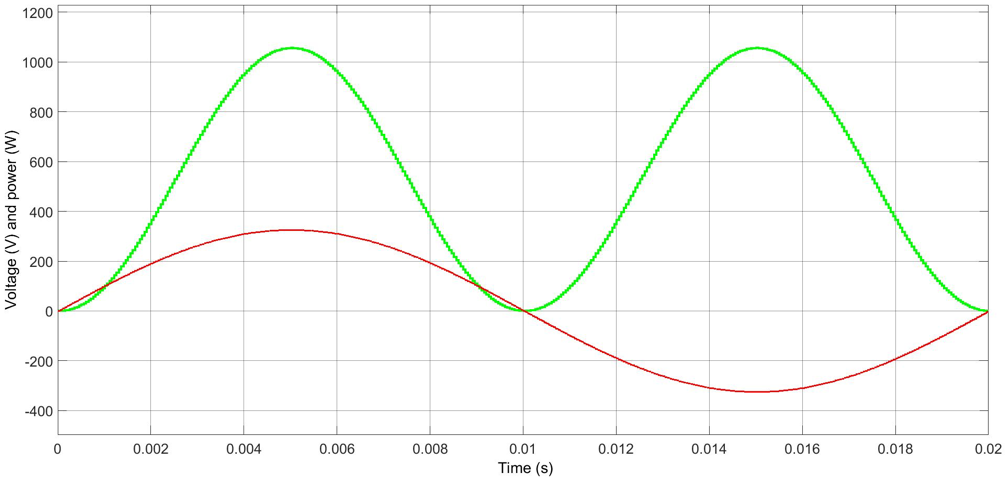# Basics of reactive power compensation (Part 1)

Power is defined as a rate of flow of energy past a given point. In electrical circuits, power is also defined as the product of instantaneous voltage and instantaneous current. In an alternating current (AC) circuit the voltage oscillates between positive and negative maximum values at the frequency of the network. Consequently, in a resistive circuit also the current oscillates at the same frequency, because the current of a resistive load is directly proportional to applied voltage. As explained before, instantaneous power is defined as the product of instantaneous voltage and current. Figure 1 presents AC voltage and respective current in a resistive circuit. Figure 2 presents the instantaneous power flowing in the circuit with respect to the AC voltage.Figure 1: Voltage and current in a resistive AC circuit.Figure 2: Power of a resistive AC circuit.

From Figure 2 it can be seen how instantaneous power oscillates at twice the frequency of the supply voltage, but unlike voltage the power never goes negative. This portion of power flow that ‒ averaged over a complete cycle of the AC waveform ‒ results in net transfer of energy in one direction known as real power or active power.

Transmission and distribution systems are basically designed for transmitting active power from power stations to power users. A number of loads, however, also require reactive power for operation. For example, AC motors and modern fluorescent lighting require magnetic fields, which are generated by reactive power consuming inductors. Also all components in transmission and distribution systems having inductive reactance such as transformers, lines, etc., require reactive power.